# Introduction: What does Data2LD do?

Data2LD estimates the parameters that define a linear differential equation that models observations of one or more curves evolving over time, space or another continuum.

Below we offer a basic introduction to systems of linear differential equations, but it would be useful of obtain the text Ramsay and Hooker (2017) for additional background on linear differential equations and examples of data analyses using models of this nature.

You will need to have a working knowledge of the functional data software package fda in order to implement a Data2LD analysis. If you are a little shakey on the subject of functional data analysis, you can consult Kokowszka, P. (2017) and Ramsay, Hooker and Graves (2009) as introductory references or Ramsay and Silverman (2005) as a more complete source.

These notes are intended to only introduce the idea of a differential equation and how to set up a data analysis using such an equation as a model. The examples here are elementary. More sophisticated sample analyses are to be found in the demo files

• AverageTemp
• CruiseControl
• Fdascript
• Refinery

We won't go into the math here, but we want to explain the key idea behind how Data2LD estimates the parameters that define the differential equation. The values of these parameters are contained in a one-column matrix that we call theta in our code examples.

Actually, there are two sets of parameters that are needed to define the solution of a differential equation. Although we focus on the typically small number that define the equation itself, we also need a larger number of parameters that we use to define a variable in the differential equation, $$x(t)$$. We represent the one or more of these at computational level by choosing a set of basis functions, $$\phi_k(t), k=1,…,K$$, that is sufficiently rich that it can accommodate how complex the shape of $$x(t)$$ may be. Choosing the right basis is a key step, which we will comment on in these notes.

Each of these basis functions $$\phi_k(t)$$ is multiplied by a coefficient $$c_k$$, and the resulting terms are then added to define a linear expansion of our approximation to x(t). That is, $x(t) = \sum_k^K c_k \phi_k(t).$ Depending on how curvature and other shape features there are in the variable, the number of $$K$$ these coefficients is apt to be large, and if there are multiple variables in the a set of equations, this will be particularly so.

Our central idea is to define these coefficients as functions $$c_k(\theta)$$ of the parameters defining the equation. In this way, the linear expansion coefficients are no longer free to vary as they please, and ultimately the variables themselves are completely determined by the parameter values that we estimate or that we use along the way to the estimation.

This parameter cascading estimation strategy has two important advantages. First, it means that our fit to the data is low-dimensional in the sense that it depends only on the small fixed number of equation parameters. The fit also depends on a single constant $$0 \le \rho < 1$$ that controls how much emphasis is placed on satisfying the equation exactly.

The second advantage is that the computation is greatly speeded up and is much more stable relative to older approaches to parameter estimation. We also derive additional stability in the estimation by how we control $$\rho$$. For small $$\rho$$ values, the emphasis is strongly on fitting the data, and as such the computation tends to proceed relatively smoothly. We steadily increase $$\rho$$ towards one, as you will see below, and with each increase use as starting parameter values those computed for the previous value. As a consequence, you will see in our two examples that typically only two or three optimization steps are required for each value of $$\rho.$$ We are usually particularly interested in how well we fit the data for $$\rho$$ very close to one, when the variable(s) are very close to being exact solutions to the equation. Thus, we ultimately use values like $$\rho = 0.999$$.

## The architecture of a linear differential equation

### A single differential equation and its terms

A single linear differential equation has a derivative of order $$m$$ on the left side of the equation, which we shall denote by $$D^m x(t)$$ instead of using the older and more cumbersome notation $$d^m x/d t^m.$$ Although $$D^m x(t)$$ is a function of time or some other continuum, we will often minimize clutter in our notation by dropping “$$(t)$$” from our expressions.

Here are some simple examples that may help to clarify the structure of linear differential equation systems, and illustrate the notation that we use.

• First order unforced : $$Dx = \beta x$$
• First order with single forcing : $$Dx = \beta x + \alpha u$$
• First order with multiple forcing : $$Dx = \beta x + \alpha_1 u_1 + \ldots + \alpha_L u_L$$
• Second order unforced : $$D^2x = \beta_1 x + \beta_2 Dx$$
• Second order unforced with contribution from only x : $$D^2x = \beta_1 x$$
• Second order forced : $$D^2x = \beta_1 x + \beta_2 Dx + \alpha u$$

The right side of the equation consists of a sum of terms, each being the product of a coefficent, $$\alpha$$ or $$\beta$$, and a variable, $$x(t)$$ or $$u(t)$$.

The coefficient or rate may be a constant, or it may be a function of time and/or of some variable that is external to the system of equations. What the coefficient or rate function multiplies can be one of two things. If it multiplies a derivative of the variable $$x(t)$$ in the equation, we call the product a homogeneous term in the equation, and we will use the notation $$\beta$$ or $$\alpha(t)$$ for the coefficient. The homogeneous coefficient or multiplier functions $$\beta_j$$ can be functions of $$t$$, or of any other external variable, but the most frequent case in practice is that they are constants. If the system is linear, which we assume here, what coefficient cannot be is a function of the value $$x$$ or of any of its derivatives.

If the coefficient is a function of a system variable, or if what it multiplies is not just the simple value of a variable, the equation is nonlinear, and not an object that this package uses. A contrasting example is the differential equation $$Dx(t) = \beta*x(t)*[K - x(t)]$$, which is nonlinear because it involves the square of $$x$$. Nonlinear equations are treated in the package CollocInfer.

A coefficient may also multiply a function of time that is not a variable in the system, that is, not one of the variable derivatives $$D^j x(t)$$. The term then represents external input that modifies the behavior of the derivative of the variable $$D^m x(t)$$ on the left side. We call such a product a forcing term and we use the notaton $$\alpha$$ or $$\alpha(t)$$ for the coefficient, and $$u(t)$$ for an external function.

Often a term has a third multiplier, which is a fixed constant. This we call a factor in the term. It is especially often that the factor is -1, and in such cases it is usually understood that coefficient function is non-negative.

The general formulation for a homogeneous term on the right side of the equation is $$b \beta(t) D^j x(t)$$ where $$b$$ is a fixed known constant and $$j < m$$, such as the -1 that we used above. A forcing term is $$a \alpha(t) u_{\ell}(t)$$ where $$a$$ is the fixed factor, which is dropped if it is one.

In the simplest situation, the derivative order on the left side is $$m = 1$$, there is only a single term on the right side and $$\beta_j$$ is a constant. For example, the differential equation defining exponential decay over time is $$Dx(t) = -\beta x(t)$$ or, dropping “$$(t)$$”, $$Dx = -\beta x$$. Here the single constant parameter $$\beta > 0$$ determines the rate of decay, and is therefore called a “rate constant.” The factor is -1, as we indicated above. The time taken for the decay to be about 95% complete is $$4/\beta$$.

A simple order $$m = 2$$ example is the equation for harmonic motion $$D^2x = -\beta x$$, $$\beta > 0$$ and factor -1, the solution to which is the weighted sum of a sine and a cosine function. The frequency of period is $$T = 2 \pi / \beta$$ so that if $$\beta = 2 \pi$$ the solution oscillates once per time unit.

A slightly more complex equation is that for damped harmonic motion $$D^2x = -\beta_0 x - \beta_1 Dx, \beta_0 > 0$$. The solution combines sinusoidal behavior with an exponential evolution of the amplitude. Depending on the sign and size of the damping coefficient $$\beta_1$$, the evolution is exponential decrease or increase.

To sum up, what makes the equation linear is (1) that it is a sum of terms, and (2) each term is a product of a multiplier or rate function and the value of a variable or its derivative (homogeneous), or an external variable (forcing). Such is the jargon of dynamic modelling.

Chapter 3 of Ramsay and Hooker (2017) can be consulted for more details.

### Systems of linear differential equations

A system of differential equations involves $$d$$ variables $$x_i, i=1,\ldots,d$$. We allow the left side of equation $$i$$ to have an order of derivative $$m_i$$ that varies from one equation to another.

The right side of the $i$th equation is a sum of terms involving derivatives $$D^k x_j$$ of orders $$k$$ less than $$m_j$$. Although there is the potential for a very large number of such terms if $$d$$ is at all large, this usually does not happen. Rather the terms for equation $$i$$ involve only one or two variables other than the variable $$x_i$$ on the left side of the equation, as well as terms involving that variable itself.

## More example systems

All these examples involve only a single variable, and we want to extend them to allow for more than one variable with mutual additive effects on each other. Now we will need two subscripts on each coefficient, the first of which pertains to the variable being affected, and the second to the variable producing the effect. In fact, for higher order equations, we will also need a third index that specifies the order of derivative.

• Two first orders unforced : $$Dx_1 = \beta_{11} x_1 + \beta_{12} x_2$$ : $$Dx_2 = \beta_{21} x_1 + \beta_{22} x_2$$
• Two first orders with single forcing : $$Dx_1 = \beta_{11} x_1 + \beta_{12} x_2 + \alpha_{11} u_{11}$$ : $$Dx_2 = \beta_{21} x_1 + \beta_{22} x_2 + \alpha_{21} u_{21}$$
• A first order and a second order unforced : $$Dx_1 = \beta_{11} x_1 + \beta_{12} x_2$$ : $$D^2x_2 = \beta_{21} x_1 + \beta_{220} x_2 + \beta_{221} Dx_2$$ Notice in the third example that, because variable $$x_2$$ has a second order equation, there can be a contribution from the variable itself (that is, $$D^0 x_2$$) as well as from its first derivative. The third index can take values from 0 to $$m-1.$$

But, thankfully, most systems used in practice will not need this level of complexity. In fact, typically, the majority of these contributions are not in the equation at all. Moreover, some of the elements in the equation will be associated with known rate functions, for example, with values that may have been determined by earlier experiments.

Often a specific rate function $$\beta$$ or forcing function coefficient function $$\alpha$$ may appear more than once in a system of equations, in which case the notation for specifying the equations may have to be modified so as to make this clear. But it's still important to keep the three-index notation $$\beta_{i_1,i_2,j}$$ in mind since, in summary, on the right side of equation $$i_1$$ there is the potential to have a contribution from the $j$th derivative of variable $$i_2$$, as well as from forcing functions $$u_{i_1 \ell}, \ell = 1, \ldots, L_{i_1}$$ modulated by multiplicative coefficient $$\alpha_{i_1 \ell}$$.

Here, in summary, are the facets of a linear differential equation system that an Data2LD analysis can handle:

• single or multiple equations
• first order or higher order
• homogeneous or forced equations
• constant or time-varying coefficients

Future versions of Data2LD will allow other possibilities, such as multiple observations at times, and smoothing or regularization of estimated rate or coefficient functions.

## Functional observations

A single functional observation is a set of $$n$$ values $$y_j$$ at a set of time points $$t_j$$ contained with some interval that we shall assume, without losing any generality, ranges from 0 to $$T$$. For simplicity, we shall also assume that the observations are univariate, that is, each $$y_j$$ is a single real number. We also assume that the times are strictly ordered, so that $$t_j < t_{j+1}$$. What makes the observations functional is that a smooth function $$x(t)$$ underlies these data, and that $$y_j$$ reflects the function value $$x(t_j)$$.

Data2LD implicitly assumes the classic relation $$y_j = x(t_j) + e_j$$ where the “errors” or “residuals” have a distribution with mean $$\mu = 0$$, variance $$\sigma^2$$ that is finite, and that their distribution is independent of that of the $$x(t_j)$$'s. These assumptions justify the use of least squares approximation to the data, which Data2LD implements.

Note that not all of the $$d$$ functions $$x_i, i=1,\ldots,d$$ may be observed, a situation that is quite common in applications.

# What are the steps in a typical Data2LD analysis?

Here we take you through the steps and decisions required to execute an Data2LD analysis and explore the results. Also, you might be helped by following through the code in the next Section for either the single variable refinery data, or for the two-variable the cruise control example. The distribution of Data2LD contains a number of sample analyses that illustrate the use of Data2LD in live data analyses.

## Define the problem

This is where you set up the differential equation, perhaps the most important step of all.

Your greatest hazard will be exuberance! It is really easy to over-parameterize a model defined by a differential equation, and in the writer's experience, a large portion of published equations are just that. An over-parameterized equation is one for which certain coefficients and combinations of coefficients cannot be estimated given the data that one is using. Whether or not a model is over-parameterized will depend on the configuration of the data. For multi-equation systems it is common to have data available for only a subset of the equations. It may not be obvious which combinations of parameters are under-determined by the data, and a good deal of experimentation with alternative models may be required before the final equation is defined.

So begin with the simplest possible equation or system that you can imagine that has anything to say about the data, even if it is known to be only a caricature. Check the estimated system using methods described below to see that is well-defined by the data, and then consider only a few elements to the equation, preferably one at a time, again accompanied by careful confirmation of identifiability. You have been warned!

## Specify the observation interval, the observation times, and load the observations

The observation values and the times of observation are specified in a list array of length $$d$$, the number of variables in the system. A list array is an un-named list defined by the R code listArray = vector("list", d). A specfic $j$th element in the array is accessed by the R code listArray[[j]].

A specific member of listArray is itself a named list, with two members:

• argvals: This contains a vector of increasing observation times $$t$$.
• y: This contains the same number of observation values.

It is usual for some variables not to be observed, and the corresponding lists will be empty as a consequence. The number of spacing of times can vary from one list to another, but even if they are the same for all variables, this information is required in list corresponding to an observed variable.

It can happen that observations do not have a one-to-one correspondence with variables. For example, an observation may be of the sum of two variables. Unfortunately, function Data2LD cannot accommodate such data configurations, but this is a target for future development.

## Select one or more basis systems for representing the solution(s)

There are two classes of basis systems in an Data2LD analysis: the rate or coefficient function bases and the variable bases. The variable bases $$\phi_{ik}(t)$$ define the estimate of the solution functions $$x_i$$. Here some care and thought is required. The basis system is not determined so much by the data, as it would be in normal smoothing, but rather by what behaviour the variable must exhibit in order to allow an accurate solution to the equations. Often, for example, highly localized curvature is present and especially where a forcing function is discontinuous, as it often is. In such a condition, high knot density for a B-spline expansion is required, or even multiple knots at a known specific location. Both the cruise control and the head impact examples exhibit this problem. A good quality final choice for a variable system may require considerable experimentation.

The class of basis systems is those that define the coefficient or rate functions. These are usually functional data basis objects, and where constant coefficients are involved are constant basis functions. Normally the coefficient bases are much smaller in size than those for representing $$x_i(t)$$ since we expect a lot of curvature in the equation solution, but only mild curvature in a rate function $$\beta(t)$$. There is also a provision for coefficient functions that the user defines.

## Setting up vectors of list objects defining each term in each differential equation

Each rate or coefficient function is defined by one or more parameters. Some functions will have parameters that must be estimated by Data2LD, and some others will be defined by parameter values treated as fixed. All parameter values will need to be specified, however, since those to be estimated must have initial values that will be modified in the course of optimizing the fits to the data. It will often be the case that rate or coefficient functions are simple constants, and thus require only a single parameter value. A more general but still simple case is where the function is defined by a basis function expansion, and therefore can be considered as a functional data object (see references above for more information on functional data objects if needed).

The most general case is one in which the user defines the function by providing a function definition In this case, and if these parameters require estimation, a second function definition is also required for the partial derivative of the function with respect to the parameters that define it.

## Setting up the list vectors defining the system of equations

A vector of length $$d$$, which will call a modelList, specifies the structure of the system of $$d$$ differential equations in roughly the same way that you would write down the equations one after the other. The vector is defined by the code modelList <- vector("list", d).

Each list in modelList contains in turn list and other objects that define the essential information about the corresponding equation. These seven items are named members or fields, and are as follows, with the name appearing in bold face and description of the object following:

• XList: This is a one-dimension list array (list), with each list containing a list object that specifies a term in the equation that involves either $$x_i$$ or a derivative $$D^jx_i$$ of the variable. We call these the homogeneous terms in order to distinguish them from the terms involving forcing functions. The length of the XList list array is equal to the number of homogeneous terms in the equation.
The details of the list object in a list of XList will be specified in the next paragraph.
• FList: This is also a one-dimension list array, with each member containing a list object, to be described below, that specifies the nature of a forcing function term. If the equation does not have forcing term, an empty list NULL is used. The details of the list object in a list of Fterm will be specified in the next paragraph.
• order: a positive integer specifying the highest order derivative $$m$$ in the equation, the expression $$D^m x_i$$ typically appearing to the left of the equal sign for equation $$i$$.
• name: A character string to be used as a name for the variable. If not supplied, it defaults to x1, x2 and so on.
• weight: a positive number specifying a weight to used in defining the total fit to the data. This is required when variables differ widely in scale, as often happens. If not supplied, it defaults to one.

### The XList object

Now let's look at the list object that is contained in a list in XList.

The following six fields are required to be set up by the user:

• fun : one of:
• functional basis object of type basis
• a functional data object of type fd
• a functional parameter object of type fdPar
• a list object with these named fields:
• fd : a functional handle for the Matlab code defining the value of the function
• Dfd : a functional handle for the Matlab code defining the value of the derivative of the function with respect to its parameters
• more : any additional information required for the code defining the
function value
• parvec : a numeric vector containing the initial values of each of the parameters defining the coefficient function. If the function is a functional data object, this will be the vector of values of the coefficients defining its basis expansion. If the function is just a constant, then only a single real value is required, and this is taken as defining a constant functional data object.
• estimate : a logical or integer value indicating whether or not the function is to be treated as fixed. If an integer is supplied, zero indicates fixed and any other value indicates estimated.
• variable : This field contains an integer specifying which of the variables is involved in this term. Any of the variables are candidates, and they may appear in any order. It is usual to have a contribution from the equation's variable itself and/or one or more of its derivatives, but other variables may also appear.
• derivative : The order of the derivative of the variable in the term. This derivative order must be less than that variable's highest derivative order.
• –factor– : The fixed constant multiplier, such as -1.

Again we supply a single line command make.Xterm(funobj, parvec, estimate, variable, derivative, factor) that can do this set-up of a single homogeneous term for you.

### The FList object

The list objects contained in the lists of the FList list array specify the forcing functions and the coefficients $$\alpha(t)$$ that multiply them.

• fun : one of:
• functional basis object of type basis
• a functional data object of type fd
• a functional parameter object of type fdPar
• a list object with these named fields:
• fd : a functional handle for the Matlab code defining the value of the function
• Dfd : a functional handle for the Matlab code defining the value of the derivative of the function with respect to its parameters
• more : any additional information required for the code defining the
function value
• parvec : a numeric vector containing the initial values of each of the parameters defining the coefficient function. If the function is a functional data object, this will be the vector of values of the coefficients defining its basis expansion. If the function is just a constant, then only a single real value is required, and this is taken as defining a constant functional data object.
• estimate : a logical or integer value indicating whether or not the function is to be treated as fixed. If an integer is supplied, zero indicates fixed and any other value indicates estimated.
• Ufd : A functional data object of the fd class specifying the forcing function. If there are replications involved, this object may have the same number of replications, or it may be a single function, in which case, this function is used for all replicates.
• factor : An optional specification of a fixed constant multiplier for the term.
This is often either -1 or 1, but may be any value. It defaults to 1.

The command that will set this up automatically is make.Fterm(fun, parvec, estimate, Ufd, factor).

Finally, these two list vectors plus the other three objects name, order and weight that define a single linear differential equation must be combined into list object that is within the respective list of the over model list array that defines the complete system. A single line command that will do this for you is make.Variable(name, order, XList, FList, weight). The weight field can be set manually if you need to do so, and the remaining two fields nallXterm and nallFterm are automatically set when you use the make.Model function described below.

The function checkModel should be used immediately after the model list array is specified, and it will be called automatically within Data2LD. It can be invoked by the command checkModel(XbasisList, modelList,).

## Compute starting values for estimated coefficients using derivative matching

This is an optional step, since Data2LD often works fine with simple initial coefficient values like zero, and supplying good initial values often only saves one or two iterations.
However, if good initial values are considered important, these can be constructed by a process that we call *gradient matching.} This involves an initial smoothing of the data for each observed variable, with a view to estimating the highest required derivative. Then the derivatives are converted to functional data objects, and these are used in the arguments of the fRegress function that fits a concurrent linear model. \cite{Ramsay:09} can be consulted for further details, and the cruise control examples show how this is done in that context.

## Preliminary computations of four-way tensors in checkModel()

In this as well as in many algorithms, certain quantities need only be computed once, and once set up, can be reused each time a function is invoked. In the case of Data2LD, the integral of products of four basis functions in all pairs of terms are required over and over again, and the approximation of these integral can be a time-consuming. But this computational load can be greatly reduced by computing once and for all the four-way array of integrals of products of four basis functions, where the basis functions involved are those the $$\beta$$ and/or $$\alpha$$ bases and at the same time those of possible pairs of solution basis functions.

This is done in the checking function checkModel, and using this function before doing any analysis should always be done. In addition, the function displays a nice summary of the structure of the model as a means of further checking.

## A simple example: The refinery data

Our simplest example is the analysis of the refinery data described in our references and available for analysis in our distribution package.

The single differential equation is $$Dx(t) = -\beta x(t) + \alpha u(t)$$. Note that both rate coefficient $$\beta$$ and forcing coefficient $$\alpha$$ are constants. By convention, a minus sign appears in front of $$\beta$$, which is expected to be positive. The homogeneous part of the equation, $$Dx(t) = -\beta x(t)$$, is the differential equation for exponential decay. The data are observations of fluid level in a tray in a refinery cracking tower, and the forcing function is a valve setting which is closed until time 67 minutes when it is opened. The forcing function is specified as a functional data object Ufd, and is a step function. The observations are recorded until 193 minutes. Both coefficients will be estimated.

These commands set up the data that we want to analyze:

library("Data2LD")

## Loading required package: fda

## Loading required package: Matrix

##
## Attaching package: 'fda'

## The following object is masked from 'package:graphics':
##
##     matplot

## Loading required package: splines

## Loading required package: deSolve

## Loading required package: knitr

## Loading required package: rmarkdown

N <- 194
TimeData <- RefineryData[,1]
TrayData <- RefineryData[,2]
ValvData <- RefineryData[,3]


Now plot the data:

plot(TimeData, TrayData, type="p", xlab="Time (sec)", ylab="Speed (km/hr)")
lines(c(67,67), c(0,4.0), type="l")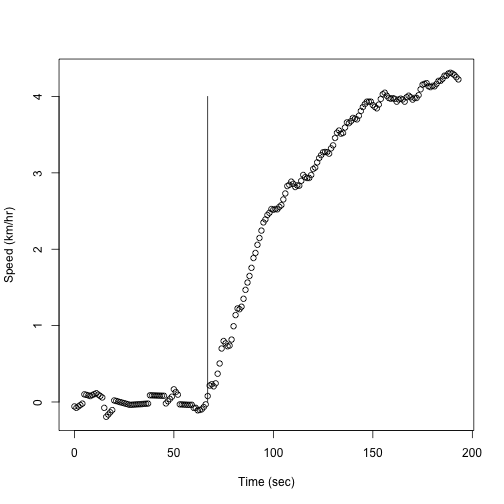plot(TimeData, ValvData, type="p", xlab="Time (sec)", ylab="Control")
lines(c(67,67), c(0,0.5), type="l")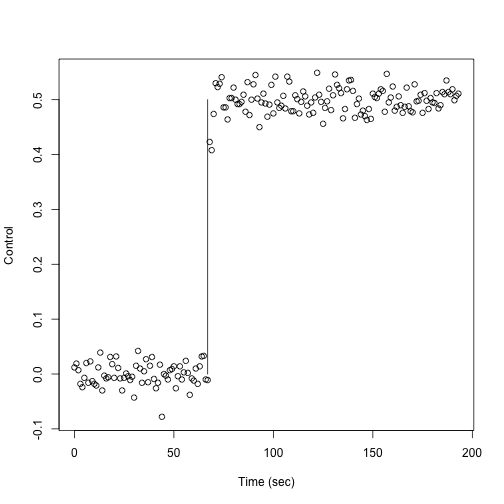We now load the observation times and the data into a list object. Here and elsewhere our un-named list objects containing named lists are of length 1. This seems unnecessary, but we stick to this format because we will often be working with more than one variable.

  TrayList <- list(argvals=RefineryData[,1], y=RefineryData[,2])
TrayDataList  <- vector("list",1)
TrayDataList[] <- TrayList


This model assumes that a single forcing function $$u(t)$$ is driving the output. This is the valve setting, which is a step function that changes its level at time 67. The following code sets up a functional data object for this forcing function $$u(t)$$.

Valvbreaks <- c(0,67,193)
Valvnbasis <- 2
Valvnorder <- 1
Valvbasis  <- create.bspline.basis(c(0,193), Valvnbasis, Valvnorder, Valvbreaks)
Valvfd     <- smooth.basis(TimeData, ValvData, Valvbasis)$fd  Here we define and plot the spline basis object that will be used to represent the solution $$x(t)$$ and load it into a list object of length one. Here a little explanation about our order and knot choices for represent $$x(t)$$ is needed.We use order 5 B-splines as a basis because we want the first derivative to be smooth nearly everywhere, except at the time 67 of the valve opening when an abrupt change in the input forcing function takes place. There we have to allow the first derivative to be continuous but not its higher derivatives. We achieve this by putting four knots at the same value, namely 67 minutes. After time 67, 13 equally spaced interior knots are used, but before then, where the function is flat, we have no interior knot.  Traynorder <- 5 Traybreaks <- c(0, rep(67,3), seq(67, 193, len=15)) Traynbasis <- 22 TrayBasis <- create.bspline.basis(c(0,193), Traynbasis, Traynorder, Traybreaks)  We plot the basis so that we can see that placing two knots or breakpoints at time 67 will leave the tray level function $$x(t)$$ continuous at 67 but will allow its first derivative to be discontinuous. The vertical dashed lines indicate knot placements.  par(mfrow=c(1,1)) plot(TrayBasis, xlab="", ylab="B-spline basis functions")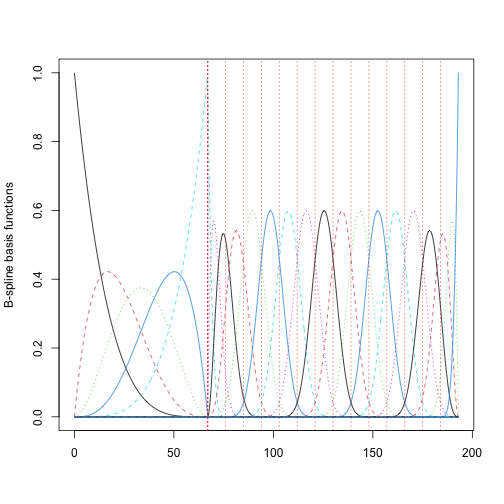TrayBasisList <- vector("list",1) TrayBasisList[] <- TrayBasis  Now we turn to defining the right side of the equation. Both of the coefficient functions are constants, but we should still define them as functional data objects in order to remind ourselves that coefficients can be functions of time. In this code we define a constant basis over the observation interval, make it into a functional data object with value zero everywhere, and then make two fdPar objects, one for the homogeneous term, and one for the forcing term. conbasis <- create.constant.basis(c(0,193)) confd <- fd(0,conbasis) betafdPar <- fdPar(confd) alphafdPar <- fdPar(confd)  This is overkill; we could just put conbasis in as the coefficient function for both terms, we do want to keep the process in its most general form. Next we define the list object of length 1 containing the specification of the single homogeneous term of the model. In the definition, we supply a reasonable positive value for the value of the coefficient function, specify that its value will be optimized in the estimation process, that it will multiple the variable itself rather than one of its derivatives, and that there will be a fixed factor -1 multiplying the term. How did we get the value 0.04? We know that an exponential decay is pretty much completed in $$4/\beta$$ time units (minutes), and from our plot of the data we could that this took about 193 - 67 = 126 minutes. Using 4/0.04 = 100 minutes is close enough in this context.  # Xterm Fields: funobj parvec estimate variable deriv. factor XTerm <- make.Xterm(betafdPar, 0.04, TRUE, 1, 0, -1) XList <- vector("list", 1) XList[] <- XTerm  Then we define the list object containing the specification of the single forcing term.  # Fterm Fields: funobj parvec estimate Ufd factor FTerm <- make.Fterm(alphafdPar, 1.0, TRUE, Valvfd, 1) FList <- vector("list", 1) FList[] <- FTerm  Now we assemble these terms into the definition of the first order forced linear differential equation.  TrayVariable <- make.Variable(XList=XList, FList=FList, name="Tray level", order=1) TrayModelList <- vector("list",1) TrayModelList[] <- TrayVariable  Finally we define the model object by combining the basis system with the variable definitions and the coefficient definitions. This step is also mandatory because it not only checks the structure of the objects in the argument list, but it also computes some four-way arrays or tensors that are used repeatedly in an analysis. It also displays the structure of the model so that we make a visual check as well. checkModelList <- checkModel(TrayBasisList, TrayModelList, summarywrd=TRUE)  ## ---------------------------------------------------------------------- ## Variable 1 Tray level , order = 1 , weight = 1 ## Homogenous term(s): ## Homogenous term 1 , variable 1 , derivative = 0 , factor = -1 ## Parameter vector position(s): ## 1 ## Parameter vector value(s): ## All values = 0.04 ## Coefficient type is functional data object ## All parameters are estimated. ## ---------------------------------------- ## Forcing terms: ## Forcing term 1 , factor = 1 ## Parameter vector position(s): ## 2 ## Parameter vector value(s): ## All values = 1 ## Coefficient type is functional data object ## All parameters are estimated. ## ----------------------------------------------------------------------  TrayModelList <- checkModelList$model
nparam         <- checkModelList$nparam print(paste("Number of parameters =",nparam))  ##  "Number of parameters = 2"  We invoke function Data2LD() once to ensure that everything is working. To do this, we must define a value of the smoothing parameter $$\rho$$ that controls the degree of smoothness in the solution function. rhoVec <- 0.5 Data2LDList <- Data2LD(TrayDataList, TrayBasisList, TrayModelList, rhoVec) MSE <- Data2LDList$MSE    # Mean squared error for fit to data
DMSE <- Data2LDList$DpMSE # gradient with respect to parameter values  We can now proceed to the optimizations of data fits for a sequence of values of $$\rho$$. The following code sets up values that control the optimization, and defines a sequence of $$\rho$$ values: dbglev <- 1 # debugging level iterlim <- 50 # maximum number of iterations convrg <- 1e-6 # convergence criterion gammavec <- 0:7 nrho <- length(gammavec) rhoMat <- as.matrix(exp(gammavec)/(1+exp(gammavec)),nrho,1) dfesave <- matrix(0,nrho,1) gcvsave <- matrix(0,nrho,1) MSEsave <- matrix(0,nrho,1) ISEsave <- matrix(0,nrho,1) thesave <- matrix(0,nrho,nparam)  The value of $$\rho$$ provided by the argument rho requires special comment, and a more detailed explanation of its meaning can be found in \cite{RamsayHooker17}. the value of $$\rho$$ may be either a single number greater than or equal to zero and less than one, or a vector of such numbers. The role of these values is to control the relative emphasis on fitting the data versus satisfying the differential equation. For lower values such as 0.5, the data will be closely approximated but the resulting $$x(_i)t)$$ will not fit satisfy the differential equation particularly well, and the estimates of the parameters in theta.opt will be relatively poor. On the other hand, high values like 0.999 will place strong emphasis on fitting the differential equation and provide good parameter estimate, but at the expense of a poorer fit to the data. For smaller values of $$\rho$$ the optimization proceeds rapidly, but if we proceed directly to use large values, the optimization can become more difficult, and may terminate in a local minimum that is not globally optimal. We therefore recommend using a vector of values, and the optimization code will use these one after another, feeding the parameter estimates at each value on as initial values for the next optimization. The values of rho be increasing, but not by equal steps. Instead, using the formula $$\rho = \exp(\gamma)/[1 + \exp(\gamma)]$$ where the increasing values of $$\gamma$$ can be integer sequences such as 0, 1, …, 7, which values the values of $$\rho$$ as 0.5, 0.73, 0.88, 0.95, 0.98, 0.99, 0.997, and 0.999. Here we cycle through the increasing values of $$\rho$$ and optimize the fit using function Data2LD.opt. Note that we pass the parameter estimates at each value on as initial values for the next optimization. TrayModelList.opt <- TrayModelList for (irho in 1:nrho) { rhoi <- rhoMat[irho] print(paste("rho <- ",round(rhoi,5))) Data2LDResult <- Data2LD.opt(TrayDataList, TrayBasisList, TrayModelList, rhoi, convrg, iterlim, dbglev) theta.opti <- Data2LDResult$thetastore
TrayModelList.opti <- modelVec2List(TrayModelList, theta.opti)
Data2LDList <- Data2LD(TrayDataList, TrayBasisList, TrayModelList, rhoi)
MSE       <- Data2LDList$MSE df <- Data2LDList$df
gcv       <- Data2LDList$gcv ISE <- Data2LDList$ISE
Var.theta <- Data2LDList$Var.theta thesave[irho,] <- theta.opti dfesave[irho] <- df gcvsave[irho] <- gcv MSEsave[irho] <- MSE ISEsave[irho] <- ISE }  ##  "rho <- 0.5" ## ## Iter. Criterion Grad Length ## 0 0.004056 0.002639 ## 1 0.003795 0.000929 ## 2 0.003469 0.000201 ## 3 0.003468 9e-06 ## 4 0.003468 2e-06 ## Convergence reached. ## ##  "rho <- 0.73106" ## ## Iter. Criterion Grad Length ## 0 0.006556 0.007342 ## 1 0.004993 0.007109 ## 2 0.003548 0.000709 ## 3 0.003509 0.000245 ## 4 0.003509 2.4e-05 ## Convergence reached. ## ##  "rho <- 0.8808" ## ## Iter. Criterion Grad Length ## 0 0.01936 0.042393 ## 1 0.017959 0.040905 ## 2 0.003673 0.002004 ## 3 0.003643 0.000351 ## 4 0.003643 9e-06 ## Convergence reached. ## ##  "rho <- 0.95257" ## ## Iter. Criterion Grad Length ## 0 0.086546 0.40182 ## 1 0.072464 0.122913 ## 2 0.004183 0.011347 ## 3 0.004032 0.001309 ## 4 0.004032 3.6e-05 ## Convergence reached. ## ##  "rho <- 0.98201" ## ## Iter. Criterion Grad Length ## 0 0.433217 2.267562 ## 1 0.316833 0.510165 ## 2 0.005904 0.100105 ## 3 0.00493 0.006987 ## 4 0.004914 0.000478 ## 5 0.004914 1.3e-05 ## Convergence reached. ## ##  "rho <- 0.99331" ## ## Iter. Criterion Grad Length ## 0 1.998634 4.272143 ## 1 1.111528 7.034821 ## 2 0.013226 0.501096 ## 3 0.006577 0.215662 ## 4 0.006174 0.008888 ## 5 0.006173 0.00022 ## Convergence reached. ## ##  "rho <- 0.99753" ## ## Iter. Criterion Grad Length ## 0 6.987144 69.17586 ## 1 0.425031 1.739904 ## 2 0.020105 3.878891 ## 3 0.007235 0.063182 ## 4 0.007229 0.001001 ## 5 0.007229 1.9e-05 ## Convergence reached. ## ##  "rho <- 0.99909" ## ## Iter. Criterion Grad Length ## 0 15.98707 361.3725 ## 1 0.488498 2.563965 ## 2 0.049116 10.80527 ## 3 0.008158 0.389031 ## 4 0.007833 0.018539 ## 5 0.007832 0.000107 ## Convergence reached.  We display for each value of $$\rho$$ some summary measures. Value df is a measure of degrees of freedom in the fit. As $$\rho$$ nears one, this value approaches 2, the number of parameters in the model. Value gcv is called the generalized cross-validation value, and is often used to choose the best value of $$\rho$$. Value MSE is the mean-squared error of the fit to the data, and value ISE is the integrated squared error between the left and right sides of the differential equation. print(" rho df gcv, MSE ISE")  ##  " rho df gcv, MSE ISE"  for (irho in 1:nrho) { print(round(c(rhoMat[irho,1], dfesave[irho], gcvsave[irho], MSEsave[irho], ISEsave[irho]),5)) }  ##  0.50000 21.68390 0.00514 0.00406 0.03731 ##  0.73106 20.93105 0.00824 0.00656 0.05355 ##  0.88080 19.27951 0.02387 0.01936 0.06222 ##  0.95257 16.43224 0.10331 0.08655 0.06251 ##  0.98201 12.72587 0.49618 0.43322 0.05505 ##  0.99331 8.98773 2.19753 1.99863 0.03965 ##  0.99753 5.80138 7.42455 6.98714 0.02021 ##  0.99909 3.46914 16.57455 15.98707 0.00648  As we indicated in the introduction, we often want to see how well we fit the data using a nearly exact solution to the equation. This code evaluates that fit at the highest value $$\rho = 0.999.$$ irho <- nrho # evaluate solution for (highest rho value irho <- 8 # evaluate solution for (highest rho value TrayModelList <- modelVec2List(TrayModelList, thesave[irho,]) Data2LDList <- Data2LD(TrayDataList, TrayBasisList, TrayModelList, rhoMat[irho,]) df <- Data2LDList$df
gcv       <- Data2LDList$gcv MSE <- Data2LDList$MSE
ISE       <- Data2LDList$ISE Var.theta <- Data2LDList$Var.theta
beta  <- thesave[irho,1]
alpha <- thesave[irho,2]
print(paste("beta  =",round(beta,3)))

##  "beta  = 0.023"

print(paste("alpha =",round(alpha,3)))

##  "alpha = 0.208"

print(paste("df  = ", round(df,1)))

##  "df  =  4"

print(paste("gcv = ", round(gcv,5)))

##  "gcv =  0.00817"

print(paste("MSE = ", round(MSE,5)))

##  "MSE =  0.00783"

print(paste("ISE = ", round(ISE,5)))

##  "ISE =  0"


This code plots the fit to the tray level data along with the data.

# plot fit of solution to data
Trayfd   <- Data2LDList$XfdParList[]$fd
tfine    <- seq(0,193,len=101)
Trayfine <- eval.fd(tfine, Trayfd)
Ufine    <- eval.fd(tfine, Valvfd)
par(mfrow=c(1,1))
plot(tfine, Trayfine, type="l", lwd=2,
xlab="Time", ylab="Tray level", xlim=c(0,193), ylim=c(-0.5,4.5))
points(TimeData, TrayData)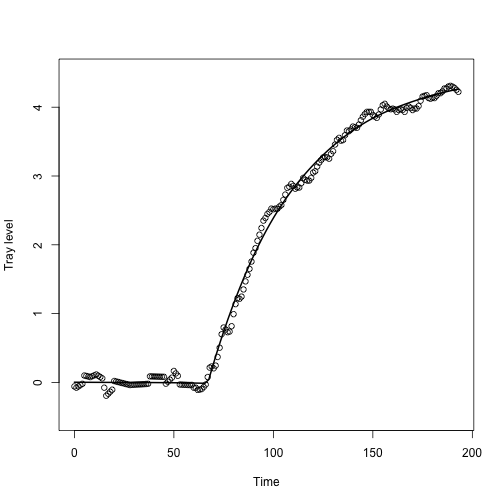We have estimated the two constant coefficients in the homogeneous and forcing terms. How well does the data define our estimated values? This requires estimates of standard errors of these estimates, which we can easily extract from the matrix Var.theta returned by function Data2LD using the highest value of $$\rho$$.

theta   <- thesave[nrho,]
stderr  <- sqrt(diag(Var.theta))
thetaUP <- theta + 2*stderr

##  0.0228 0.0217 0.0238 0.0005

print(round(c(theta, thetaDN, thetaUP, stderr),4))

##  0.2080 0.2013 0.2147 0.0034


At the highest value of $$\rho$$ we hope (but cannot guarantee) that the linear differential equation will be almost exactly solved by the function fitting the data. If so, and if we are satisfied with the fit to the data, then we can celebrate having found the right model for the data. In the following code, we compute values of the left and right side of the equation and display then plotted over each other.

# compute the derivative Dx(t) and the right side of the equation
theta <- thesave[irho,]
DTrayData <- eval.fd(TimeData, Trayfd, 1)
DTrayfit  <- -theta*Trayfine + theta*Ufine
# plot the left and right sides of the equation
plot(TimeData, DTrayData, type="b", lwd=2,
xlab="Time", ylab="DTray level", xlim=c(0,193), ylim=c(-0.01,0.12))
lines(tfine, DTrayfit)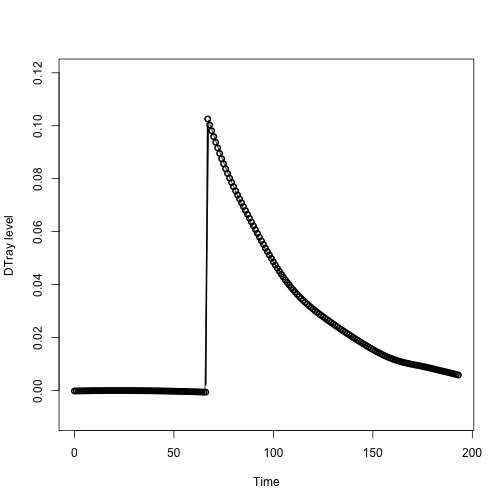We see that at the highest value of $$\rho$$ we have exactly satisfied the differential equation since first derivative values (circles), estimated by smoothing the data and the fit (smooth curve) nearly overlap.

What have we achieved here? This simplest of differential equations is able to capture how tray level responds to a change in valve setting by using only two parameters. The fit to the data is about as good as we could wish.

Further details are available in both Ramsay and Hooker (2007) and in the code that can be invoked by the command demo("Refinery").

## A two-variable system: The cruise control model

This example is not much more complicated, but does illustrate how one rate function can appear in more than one place in a system. It models how a simple feedback system works, in this case the accelerator pedal in a car. The two variables are the speed ($$S$$) of a car and feedback ($$C$$) as the driver manipulates the accelerometer to change the target speed ($$S_0$$). The data consist of 41 simulated observations for each variable, distributed uniformly over the time interval [0,80]. The input variable is the desired speed or set point $$S_0(t)$$, and it is changed at times 0, 20, 40 and 60 to speeds 60, 40, 80 and 60 kilometres per hour, respectively.

The two differential equations are: $DS(t) = -\beta_{SS} S(t) + \beta_{SC} C(t)$ $DC(t) = \beta_{CS} S(t) - \beta_{CC} C(t) + \alpha_{C} S_0(t)$ where $$\beta_{SS} = 1$$, $$\beta_{SC} = ¼$$, $$\beta_{CS} = -1$$, $$\beta_{CC} = 0$$ and $$\alpha_C = 1.$$ The homogeneous part of the speed equation is again that of exponential decay, and it is forced by the control variable. The homogeneous part of the control equation is held fixed at zero, and it is forced by the difference between the set point and the current speed. The system works by increasing $$C$$ when the current speed is less than the target and decreasing it when it is greater. The increase is passed into the speed equation as an additive forcing by $$C$$ modulated by the value of $$\alpha_S$$. Because of the exponential decay dynamics of $$S$$, the speed will approach the new speed target with an exponentially decreasing rate.

T     <- 80  #  seconds
rng   <- c(0,T)
n     <- 41
tobs  <- seq(0,T,len=n)
tfine <- seq(0,T,len=501)


We now set up the set-point forcing function. The set-point function uses an order 1 step function B-spline basis in order to define a function that is piecewise constant, since order 1 implies that there is only one parameter defining each basis function. The knots are placed at the points where the set-point changes values.

steporder  <- 1  #  step function basis
stepnbasis <- 4  #  four basis functions
stepbreaks <- c(0,20,40,60,80)
stepbasis  <- create.bspline.basis(rng, stepnbasis, steporder, stepbreaks)
stepcoef   <- c(60,40,80,60)  # target speed for each step
SetPtfd    <- fd(stepcoef, stepbasis)  # define the set point function


In this example we simulate data, and this will require that we construct the true solutions to the two equations, and then add some noise to simulate data with error.

In order to compute the solutions, we first define a function that evaluates the right side of the equation for a time value:

cruise0 <- function(t, y, parms) {
DSvec    <- matrix(0,2,1)
Uvec     <- eval.fd(t, parms$SetPtfd) DSvec <- -y + y/4 DSvec <- Uvec - y return(list(DSvec=DSvec)) }  We first have a look at the solution at a fine mesh of values by solving the equation for the points in tfine using the differential equation solver lsoda in the deSolve package. We confess we ran into a small glitch here. If we solved the equation of the full interval $$0,80$$, lsoda tried to solve equation for a time value slightly larger than 80. So we solved the equation for all but last time value, and then tacked on the true solution values at the end. The matrix ytrue that is returned has the time values in the first column and the values of the speed and control variables in the remaining two columns y0 <- matrix(0,2,1) parms = list(SetPtfd=SetPtfd) # define the input to the cruise0 function above ytrue = lsoda(y0, tfine[1:500], cruise0, parms) # solve the equation using an initial value solver ytrue = rbind(ytrue,matrix(c(80,60,240),1,3)) # combine with the final values  Now we use the interpolation function approx to get true values at the 41 observation times. speedObs <- approx(tfine, ytrue[,2], seq(0,80,len=41))$y
controlObs  <- approx(tfine, ytrue[,3], seq(0,80,len=41))$y  Next we simulate noisy data at the observation points by adding a random zero-mean Gaussian deviate to each curve value. The deviates for speed have a standard deviation 2 kilometers per hour, and those for the control level have a standard deviation of eight control units. sigerr <- 2 yobs <- matrix(0,length(tobs),2) yobs[,1] <- as.matrix( speedObs + rnorm(41)*sigerr) yobs[,2] <- as.matrix(controlObs + rnorm(41)*sigerr*4)  Now plot the data along with the true solution: plot(tfine, ytrue[,2], type="l", xlab="Time (secs)", ylab="Speed") lines(c(0,T), c(60,60), lty=3) points(tobs, yobs[,1], pch="o")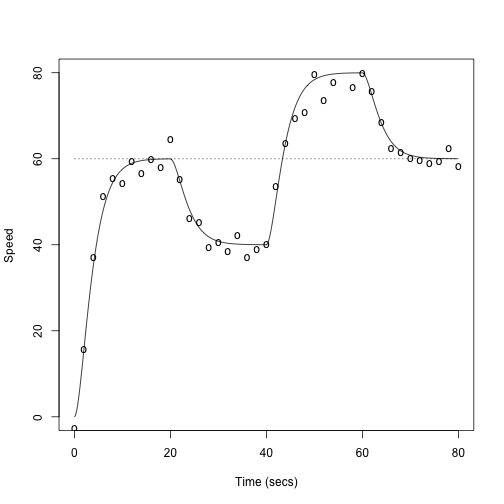plot(tfine, ytrue[,3], type="l", xlab="Time (secs)", ylab="Control level") lines(c(0,T), c(240,240), lty=3) points(tobs, yobs[,2], pch="o")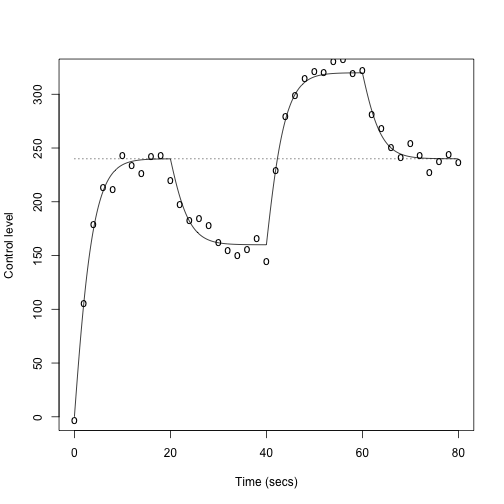We now provide simulated noisy data values in a list vector of length 2 observed for the values in tobs, and also the true values over the fine mesh tfine. Sdata <- list(argvals=tobs, y=yobs[,1]) Cdata <- list(argvals=tobs, y=yobs[,2]) cruiseDataList <- vector("list",2) cruiseDataList[] <- Sdata cruiseDataList[] <- Cdata  The next step is to set up basis objects for the values of the two variables. The acceleraton of the speed variable will be smooth but the acceleration of the control variable will change discontinuously at the points where the forcing function changes values in a stepwise fashion. For this reason the speed B-spline basis is of order five but the control variable is of order four. We use three knots at the points of input change in order to capture these degrees of smoothness. We also position a single knot between each interval between change points. cruiseBasisList <- vector("list",2) delta <- 2*(1:10) breaks <- c(0, delta, 20, 20+delta, 40, 40+delta, 60, 60+delta) nbreaks <- length(breaks) nSorder <- 5 nSbasis <- nbreaks + nSorder - 2 Sbasis <- create.bspline.basis(c(0,80), nSbasis, nSorder, breaks) cruiseBasisList[] <- Sbasis nCorder <- 4 nCbasis <- nbreaks + nCorder - 2 Cbasis <- create.bspline.basis(c(0,80), nCbasis, nCorder, breaks) cruiseBasisList[] <- Cbasis  Now that we have the data and the bases for representing the solution, we can turn to the task of specifing the five constant coefficient functions $$\beta_{SS}, \beta_{SC}, \beta_{CS}, \beta_{CC}$$ and $$\alpha_C$$. Here we use the handy functions make.Xterm and make.Fterm to set up each rate or forcing coefficient. We make the homogeneous term $$\beta_C$$ zero and not estimated, leaving only four parameters to be estimated from the data. First set up a functional parameter object that has the constant value 1 over all the interval. This defines each of the coefficient functions. conbasis <- create.constant.basis(rng) confdPar <- fdPar(conbasis)  There are two homogeneous terms for each equation, and the second equation has a forcing function which sets the target speeds. Here we define each of the five terms. # List object for the speed equation: A term for speed and a term for control, but no forcing SList.XList = vector("list",2) # Fields: funobj parvec estimate variable deriv. factor SList.XList[] <- make.Xterm(confdPar, 1, TRUE, 1, 0, -1) SList.XList[] <- make.Xterm(confdPar, 1/4, TRUE, 2, 0, 1) SList.FList = NULL SList = make.Variable("Speed", 1, SList.XList, SList.FList) # List object for the control equation: a term for speed, and a zero-multiplied term for control plus a term for the forcing function SetPtfd CList.XList <- vector("list",2) # Fields: funobj parvec estimate variable deriv. factor CList.XList[] <- make.Xterm(confdPar, 1, TRUE, 1, 0, -1) CList.XList[] <- make.Xterm(confdPar, 0, FALSE, 2, 0, 1) CList.FList <- vector("list",1) # Fields: funobj parvec estimate Ufd factor CList.FList[] <- make.Fterm(confdPar, 1, TRUE, SetPtfd, 1) CList <- make.Variable("Control", 1, CList.XList, CList.FList) # List array for the whole system cruiseVariableList <- vector("list",2) cruiseVariableList[] <- SList cruiseVariableList[] <- CList  Now set up the model object. cruiseModelList <- vector("list",2) cruiseModelList[] <- SList cruiseModelList[] <- CList  We run a check on the set up of the model. It is so easy to slip up somewhere! cruiseModelCheckList <- checkModel(cruiseBasisList, cruiseModelList)  ## ---------------------------------------------------------------------- ## Variable 1 Speed , order = 1 , weight = 1 ## Homogenous term(s): ## Homogenous term 1 , variable 1 , derivative = 0 , factor = -1 ## Parameter vector position(s): ## 1 ## Parameter vector value(s): ## All values = 1 ## Coefficient type is functional data object ## All parameters are estimated. ## Homogenous term 2 , variable 2 , derivative = 0 , factor = 1 ## Parameter vector position(s): ## 2 ## Parameter vector value(s): ## All values = 0.25 ## Coefficient type is functional data object ## All parameters are estimated. ## ---------------------------------------------------------------------- ## Variable 2 Control , order = 1 , weight = 1 ## Homogenous term(s): ## Homogenous term 1 , variable 1 , derivative = 0 , factor = -1 ## Parameter vector position(s): ## 3 ## Parameter vector value(s): ## All values = 1 ## Coefficient type is functional data object ## All parameters are estimated. ## Homogenous term 2 , variable 2 , derivative = 0 , factor = 1 ## Parameter vector position(s): ## 4 ## Parameter vector value(s): ## All values = 0 ## Coefficient type is functional data object ## All parameters are fixed. ## ---------------------------------------- ## Forcing terms: ## Forcing term 1 , factor = 1 ## Parameter vector position(s): ## 5 ## Parameter vector value(s): ## All values = 1 ## Coefficient type is functional data object ## All parameters are estimated. ## ----------------------------------------------------------------------  cruiseModelList <- cruiseModelCheckList$modelList
nparam <- cruiseModelCheckList$nparam print(paste("total number of parameters = ", nparam))  ##  "total number of parameters = 5"  We do a preliminary evaluation of the least squares criterion at the parameter values that we have set up in coefList. Even after running the check above, it's possible that there is an error that the checking function did not catch. rhoVec <- 0.5*matrix(1,1,2) Data2LDList <- Data2LD(cruiseDataList, cruiseBasisList, cruiseModelList, rhoVec) print(Data2LDList$MSE)

##  9.694541

print(Data2LDList$DpMSE)  ## [,1] ## [1,] -14.70623 ## [2,] 65.86151 ## [3,] -18.14601 ## [4,] 0.00000 ## [5,] 11.15242  Notice that the gradient for the fourth parameter is exactly 0, so that the parameter never changes value. We are now ready to optimize the criterion with respect to parameter values. This involves the following call to function Data2LD.opt: Data2LD.opt(yList, XbasisList, cruiseList, coefList.opt, rho). Here is a setup of this nature. conv <- 1e-4 iterlim <- 20 dbglev <- 1 Gvec <- c(0:7) nrho <- length(Gvec) rhoMat <- matrix(exp(Gvec)/(1+exp(Gvec)),nrho,2) dfesave <- matrix(0,nrho,1) gcvsave <- matrix(0,nrho,1) MSEsave <- matrix(0,nrho,2) thesave <- matrix(0,nrho,nparam)  Here are the analyses for each value of $$\rho.$$ Note the we start by defining a copy of the model List, so that the optimal value estimated for any value of $$\rho$$ can be passed on as the initial value to be used in the optimization for the next $$\rho$$ value. You will now see how quickly the solution is achieved for each value of $$\rho$$. cruiseModelList.opt <- cruiseModelList # initialize the optimizing coefficient values for (irho in 1:nrho) { rhoVeci <- rhoMat[irho,] print(paste(" ------------------ rhoVeci <- ", round(rhoVeci,4), " ------------------")) Data2LDOptList <- Data2LD.opt(cruiseDataList, cruiseBasisList, cruiseModelList.opt, rhoVeci, conv, iterlim, dbglev) theta.opti <- Data2LDOptList$theta  # optimal parameter values
cruiseModelList.opt <- modelVec2List(cruiseModelList, theta.opti)
#  evaluate fit at optimal values and store the results
Data2LDList    <- Data2LD(cruiseDataList, cruiseBasisList, cruiseModelList.opt, rhoVeci,
TRUE)
thesave[irho,]  <- theta.opti
dfesave[irho]   <- Data2LDList$df gcvsave[irho] <- Data2LDList$gcv
x1fd            <- Data2LDList$XfdParList[]$fd
x1vec           <- eval.fd(tobs,  x1fd)
msex1           <- mean((x1vec - speedObs)^2)
x2fd            <- Data2LDList$XfdParList[]$fd
x2vec           <- eval.fd(tobs,  x2fd)
msex2           <- mean((x2vec - controlObs)^2)
MSEsave[irho,1] <- msex1
MSEsave[irho,2] <- msex2
}

##  " ------------------  rhoVeci <-  0.5  ------------------"
##
## 0        9.694541      31.64701
## 1        9.542768      2.77815
## 2        8.628257      6.629526
## 3        8.607744      2.107083
## 4        8.606008      0.349097
## 5        8.605913      0.112305
## Convergence reached.
##
##  " ------------------  rhoVeci <-  0.7311  ------------------"
##
## 0        16.84507      4.343713
## 1        16.84265      1.572869
## 2        16.84047      0.331089
## 3        16.84035      0.071991
## 4        16.84035      0.014824
## Convergence reached.
##
##  " ------------------  rhoVeci <-  0.8808  ------------------"
##
## 0        29.85304      52.20763
## 1        29.77158      3.487795
## 2        29.57189      0.841129
## 3        29.52954      2.937731
## 4        29.52181      0.106939
## 5        29.52042      0.131588
## 6        29.52017      0.025579
## 7        29.52012      0.025229
## Convergence reached.
##
##  " ------------------  rhoVeci <-  0.9526  ------------------"
##
## 0        48.17922      118.6845
## 1        48.08537      23.1741
## 2        44.33725      9.982827
## 3        44.13228      7.231926
## 4        44.12916      0.66348
## 5        44.12912      0.080493
## Convergence reached.
##
##  " ------------------  rhoVeci <-  0.982  ------------------"
##
## 0        56.96313      58.70684
## 1        56.95328      20.79706
## 2        55.90706      6.001991
## 3        55.90207      0.047783
## 4        55.90207      0.006524
## Convergence reached.
##
##  " ------------------  rhoVeci <-  0.9933  ------------------"
##
## 0        63.57418      17.707
## 1        63.57349      9.715813
## 2        63.31632      8.714971
## 3        63.31596      0.026088
## 4        63.31596      0.00287
## Convergence reached.
##
##  " ------------------  rhoVeci <-  0.9975  ------------------"
##
## 0        67.04695      3.292808
## 1        67.04687      6.839726
## Convergence reached.
##
##  " ------------------  rhoVeci <-  0.9991  ------------------"
##
## 0        69.24817      9.307033
## 1        69.248      6.093843
## 2        69.16991      4.512636
## 3        69.16824      0.165346
## 4        69.16821      0.069679
## Convergence reached.


Here are the summary measures for these analyses. Value RMSE is the square root of the mean squared error.

ind <- 1:nrho
#  print df, gcv and MSEs
print("    rho      df        gcv        RMSE:")

##  "    rho      df        gcv        RMSE:"

print(cbind(round(rhoMat,4),  round(dfesave,1),
round(gcvsave,1), round(sqrt(MSEsave),2)))

##        [,1]   [,2] [,3] [,4] [,5] [,6]
## [1,] 0.5000 0.5000 59.2 55.5 1.88 6.35
## [2,] 0.7311 0.7311 45.5 42.5 1.78 5.49
## [3,] 0.8808 0.8808 32.9 41.1 1.46 4.38
## [4,] 0.9526 0.9526 20.3 38.9 0.98 2.72
## [5,] 0.9820 0.9820 11.4 37.7 0.90 1.68
## [6,] 0.9933 0.9933  6.1 36.9 0.90 1.34
## [7,] 0.9975 0.9975  3.7 36.7 1.02 1.31
## [8,] 0.9991 0.9991  2.6 36.9 1.35 1.37


It can be interesting to see how the estimated parameters change over the sequence of values of $$\rho$$, noting that the fourth fixed parameter never changes value.

matplot(rhoMat, thesave, type="b", xlab="rho", ylab="theta(rho)")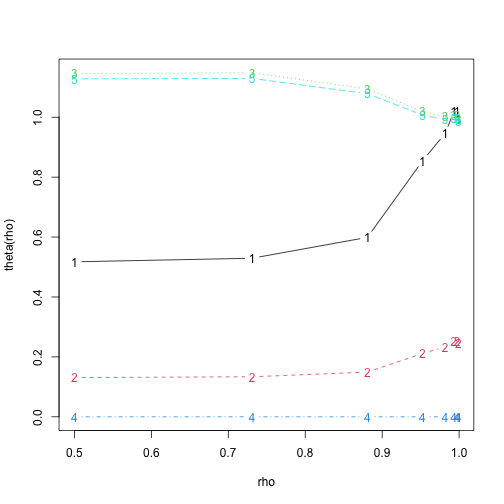Now let's look more closely at the solution for the maximum value of $$\rho$$, where the fit to the data should be also a near-solution to the differential equation.

rho.opt   <- rhoMat[nrho,]
theta.opt <- thesave[nrho,]
#  convert the optimal parameter values to optimal cruiseModelList
cruiseModelList.opt <- modelVec2List(cruiseModelList, theta.opt)
#  evaluate the solution at the optimal solution
DataLDList <- Data2LD(cruiseDataList, cruiseBasisList, cruiseModelList.opt, rho.opt,
TRUE)


Plot the estimated solutions, as estimated from the data, rather than from the initial value estimates in the above code

XfdParList <- Data2LDList$XfdParList Xfd1 <- XfdParList[]$fd
Xfd2 <- XfdParList[]$fd Xvec1 <- eval.fd(tfine, Xfd1) Xvec2 <- eval.fd(tfine, Xfd2) Uvec <- eval.fd(tfine, SetPtfd) cruiseDataList1 <- cruiseDataList[] plot(tfine, Xvec1, type="l", xlim=c(0,80), ylim=c(0,100), ylab="Speed", main=paste("RMSE =",round(sqrt(MSEsave[3,1]),4))) lines(tfine, ytrue[,2], lty=2) points(cruiseDataList1$argvals, cruiseDataList1$y, pch="o")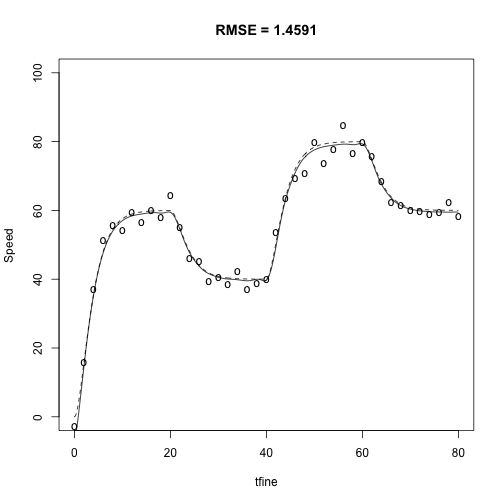#lines(tfine, Uvec, lty=2) cruiseDataList2 <- cruiseDataList[] plot(tfine, Xvec2, type="l", xlim=c(0,80), ylim=c(0,400), xlab="Time (sec)", ylab="Control", main=paste("RMSE =",round(sqrt(MSEsave[3,2]),4))) lines(tfine, ytrue[,3], lty=2) points(cruiseDataList2$argvals, cruiseDataList2$y, pch="o")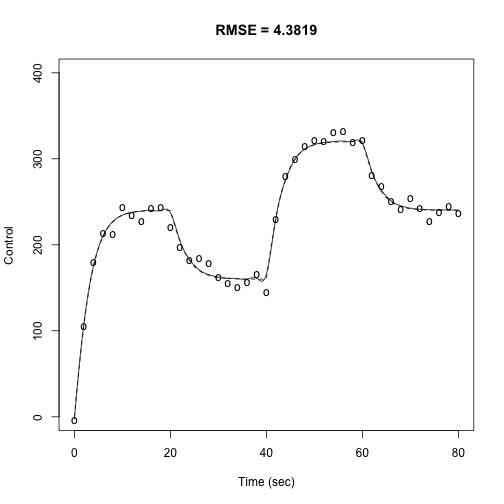Display parameters with 95% confidence limits stddev.opt <- sqrt(diag(DataLDList$Var.theta))
theta.tru <- c(1, 1/4,  1, 0,  1)
print("    True      Est.      Std. Err. Low CI    Upr CI:")

##  "    True      Est.      Std. Err. Low CI    Upr CI:"

for (i in 1:nparam) {
print(round(c(theta.tru[i],
theta.opt[i],
stddev.opt[i],
theta.opt[i]-2*stddev.opt[i],
theta.opt[i]+2*stddev.opt[i]), 4))
}

##  1.0000 0.9943 0.1549 0.6845 1.3040
##  0.2500 0.2459 0.0382 0.1695 0.3222
##  1.0000 0.9937 0.0470 0.8996 1.0878
##  0 0 0 0 0
##  1.0000 0.9849 0.0430 0.8989 1.0709


### Fitting the constrained cruise control model $$\beta_{CS} = \alpha$$

In our first analysis we ignored the fact that that the speed coefficents $$\beta_{CS}$$ and $$\alpha$$ are identical since they define a difference. Thus the four-parameter model over-fits the data by one degree of freedom. We saw that these two values were close, but not identical. Now we re-analyze the data by incorporating the constraint into the analysis, and in this way reduce the number of parameters to be estimated to 3.

Suppose that we have $$n$$ parameters and these are subject to $$k$$ constraints. We can write this in matrix terms as $$A'p = 0$$ where $$A$$ is an $$n$$ by $$k$$ matrix, each column of which defines a constraint on the $$n$$ parameter values in column vector $$p$$.

We deal with linear parameter constraints in the optmization by defining an $$n$$ by $$n-k$$ matrix $$P$$ in such a way that $$q = P'p$$ is a vector of length $$n-k$$ whose values are unconstrained, and that $$p = P*q$$ is a vector length $$n$$ that satisfies the $$k$$ constraints.

There are several ways of defining matrix \$PR with this property, but perhaps the easiest way is to use the qr decomposition. If we evaluate statement [Q,R] = qr(A), the resulting matrix $$Q$$ is $$n$$ by $$n$$ and has orthonormal columns, so that $$Q'*Q = I$$. Matrix $$R$$, not used here, is upper triangular. Matrix $$P$$ is defined as the last $$n-k$$ columns of $$Q$$, and therefore $$P'*P = I.$$

In our example, matrix $$A$$ has a single column ($$k$$ = 1), and its values are defined by the command parMap = matrix(c(0, 0, 1, 0, -1),5,1). It is not necessary to re-define the model and run checkModel. Instead, we just add matrix parMap' as an additional argument to the call to functionData2LD.opt, so that

#  parMap = matrix(c(0, 0, 1, 0, -1),5,1)
#  Data2LDOptList <- Data2LD.opt(cruiseDataList, cruiseBasisList, cruiseModelList.opt,
#                                rhoVeci, conv, iterlim, dbglev, parMap)


If you re-run the above code, you will discover that convergence is faster for each value of $$\rho$$ and that these two values are now equal. Moreover, the estimates of the other parameters are also improved

See Ramsay and Hooker (2017) for much more explanation of these analyses.

# References

• Kokoszka, P. (2017) Introduction to Functional Data Analysis. CRC Press.
• Ramsay, J. O., and Silverman, B. W.. (2005) Functional Data Analysis. New York: Springer.
• Ramsay, J. O., Hooker, G. and Graves, S. (2009) Functional Data Analysis in R and Matlab. New York: Springer.
• Ramsay, J. O. and Hooker, G. (2017) Dynamic Data Analysis. New York: Springer.